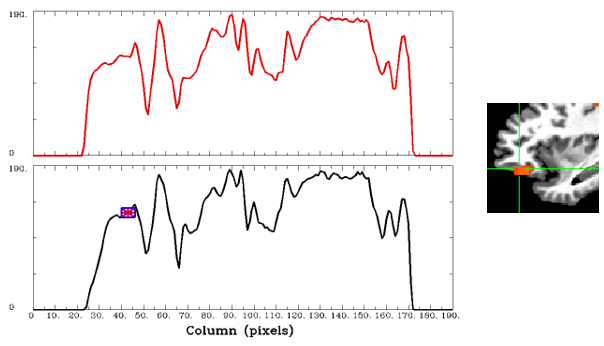# how to interpret the Rowgraphs

Hi, List experts,

I was thinking to report the activity wave for the region of my interest (ROI). I just found that in fact there is a function in the “Display”: RowGraphs. According to the help, it says, “Rowgraphs lets you graph the voxel values from image rows”. For instance, for my case, it shows two waves (one is in color, one is in black with the ROI point) -see the attached png file.

I do not know what does the x-axis “Column (pixels)” mean? What should be the y-axis? Is it “signal change”? If I report this, how should I say - it is meaningful? I was thinking that perhaps, the x-axis stands for the time (sec), but the scale 0-200 is confusing.

Thank you very much,
JHi, Juan-

A volume contains a 3D matrix of numbers, indexed with (i,j,k), which might correspond to (x,y,z) coordinates in physical units. When this dataset is viewed in the GUI, you see the numbers of the dataset-- that is, the value at each (i,j,k) location-- mapped to a color. For the underlay data, this is typically a grayscale color: for some MIN and MAX values, everything at or below MIN is black; everything at or above MAX is white, and everything in between is a scaled shade of gray.

In each image window, you are seeing one 2D plane of data: axial is the (x,y) plane (so, the (i,j) indices here); sagittal is the (y,z) plane (so, the (j,k) indices); etc.

So, you clicked “Disp” on a viewer window, and then changed the “RowGraphs” menu item from 0 → 2. What is displayed is a graph plot of the actual number values in the data set, for a 1D line of data in the given graph viewer plane; with no other options set, you are seeing the numerical values fo the dataset for the row where your crosshairs are. As you move left->right in your RowGraph window, you are moving left->right in your AFNI imageviewer window; basically, moving across the columns. That is why the x-axis label is “Column (pixels)”-- it is the integer value of the index of the columns (for a coronal or sagittal window, for example, that would be the ‘k’ index).

You have 2 graphs in your attached image; so that is why I think you selected the menu item to to be “2”; it is showing you the plot of values in your dataset in the line where your crosshair sits, as well as the line above it. The blue rectangle with a red asterisk in the middle is showing you on your (lower) graph where the crosshair is in that row of data.

–pt

I now see - thank you very much!!! Very professional!